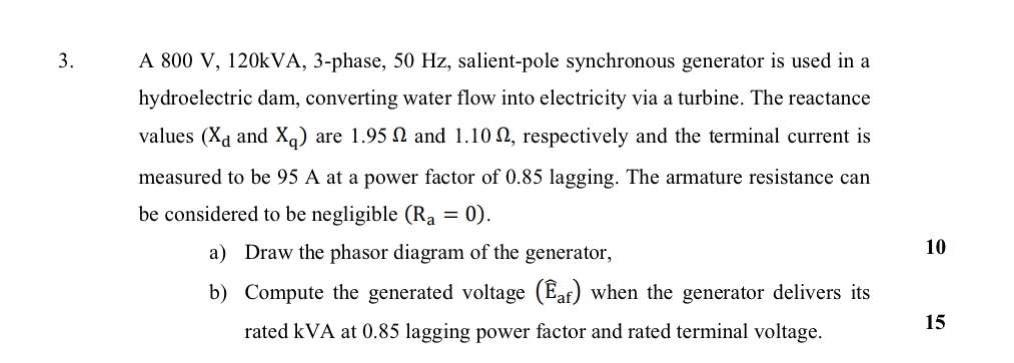# Question Solved1 AnswerThe answer for (b) should be : Generatedvoltage Eaf = 578.05 exp(j9.75°) 3. A 800 V, 120kVA, 3-phase, 50 Hz, salient-pole synchronous generator is used in a hydroelectric dam, converting water flow into electricity via a turbine. The reactance values (Xd and XQ) are 1.95 1 and 1.102, respectively and the terminal current is measured to be 95 A at a power factor of 0.85 lagging. The armature resistance can be considered to be negligible (Rg = 0). a) Draw the phasor diagram of the generator, b) Compute the generated voltage (af) when the generator delivers its rated kVA at 0.85 lagging power factor and rated terminal voltage. 10 15OOOMO7 The Asker · Electrical EngineeringThe answer for (b) should be : Generated voltage Eaf = 578.05 exp(j9.75°)

Transcribed Image Text: 3. A 800 V, 120kVA, 3-phase, 50 Hz, salient-pole synchronous generator is used in a hydroelectric dam, converting water flow into electricity via a turbine. The reactance values (Xd and XQ) are 1.95 1 and 1.102, respectively and the terminal current is measured to be 95 A at a power factor of 0.85 lagging. The armature resistance can be considered to be negligible (Rg = 0). a) Draw the phasor diagram of the generator, b) Compute the generated voltage (af) when the generator delivers its rated kVA at 0.85 lagging power factor and rated terminal voltage. 10 15
More
Transcribed Image Text: 3. A 800 V, 120kVA, 3-phase, 50 Hz, salient-pole synchronous generator is used in a hydroelectric dam, converting water flow into electricity via a turbine. The reactance values (Xd and XQ) are 1.95 1 and 1.102, respectively and the terminal current is measured to be 95 A at a power factor of 0.85 lagging. The armature resistance can be considered to be negligible (Rg = 0). a) Draw the phasor diagram of the generator, b) Compute the generated voltage (af) when the generator delivers its rated kVA at 0.85 lagging power factor and rated terminal voltage. 10 15